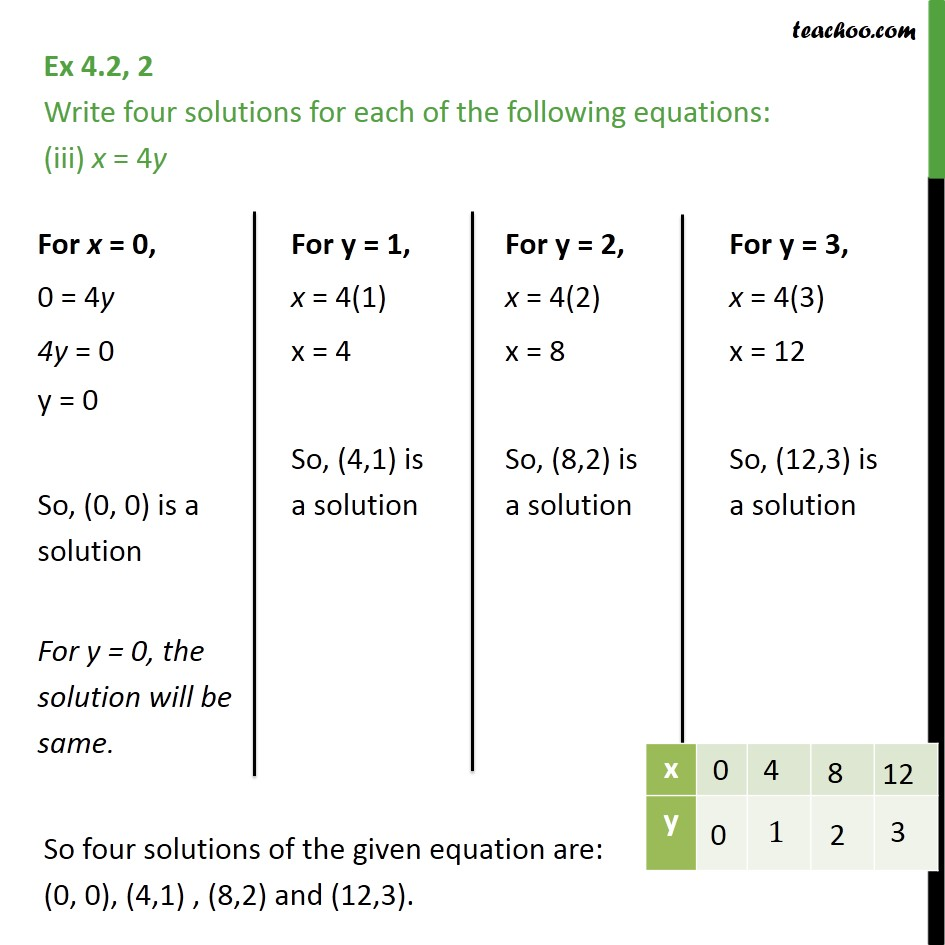1. Chapter 4 Class 9 Linear Equations in Two Variables (Term 1)
2. Serial order wise
3. Ex 4.2

Transcript

Ex 4.2, 2 Write four solutions for each of the following equations: (iii) x = 4y So four solutions of the given equation are: (0, 0), (4,1) , (8,2) and (12,3).